# pheatmap function in R

Raivo Kolde

## The `pheatmap` function

The `pheatmap` function is similar to the default base R heatmap, but provides more control over the resulting plot. You can pass a numeric matrix containing the values to be plotted.

``````# install.packages("pheatmap")
library(pheatmap)

# Data
set.seed(8)
m <- matrix(rnorm(200), 10, 10)
colnames(m) <- paste("Col", 1:10)
rownames(m) <- paste("Row", 1:10)

# Heat map
pheatmap(m)``````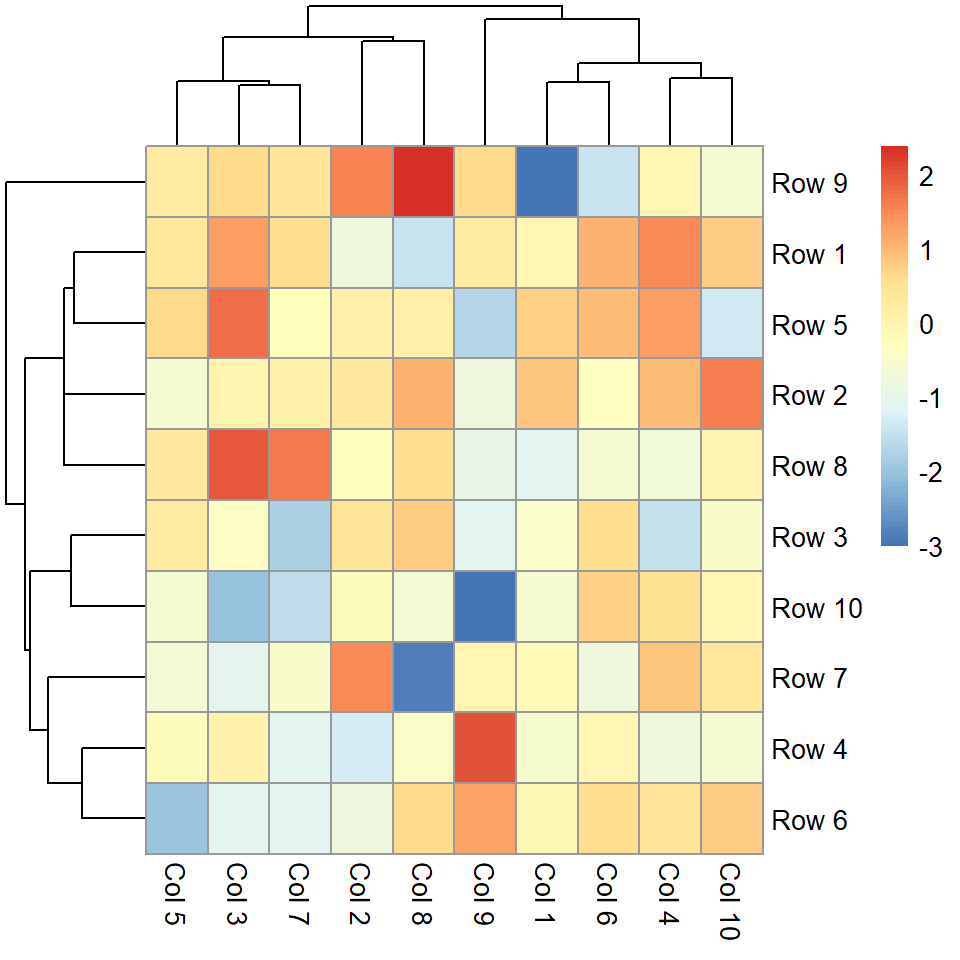Normalization

If the values of the matrix are not normalized you can normalize them by rows (`"row"`) or by columns (`"column"`) making use of the `scale` argument.

``````# install.packages("pheatmap")
library(pheatmap)

# Data
set.seed(8)
m <- matrix(rnorm(200), 10, 10)
colnames(m) <- paste("Col", 1:10)
rownames(m) <- paste("Row", 1:10)

# Heat map
pheatmap(m, scale = "column")``````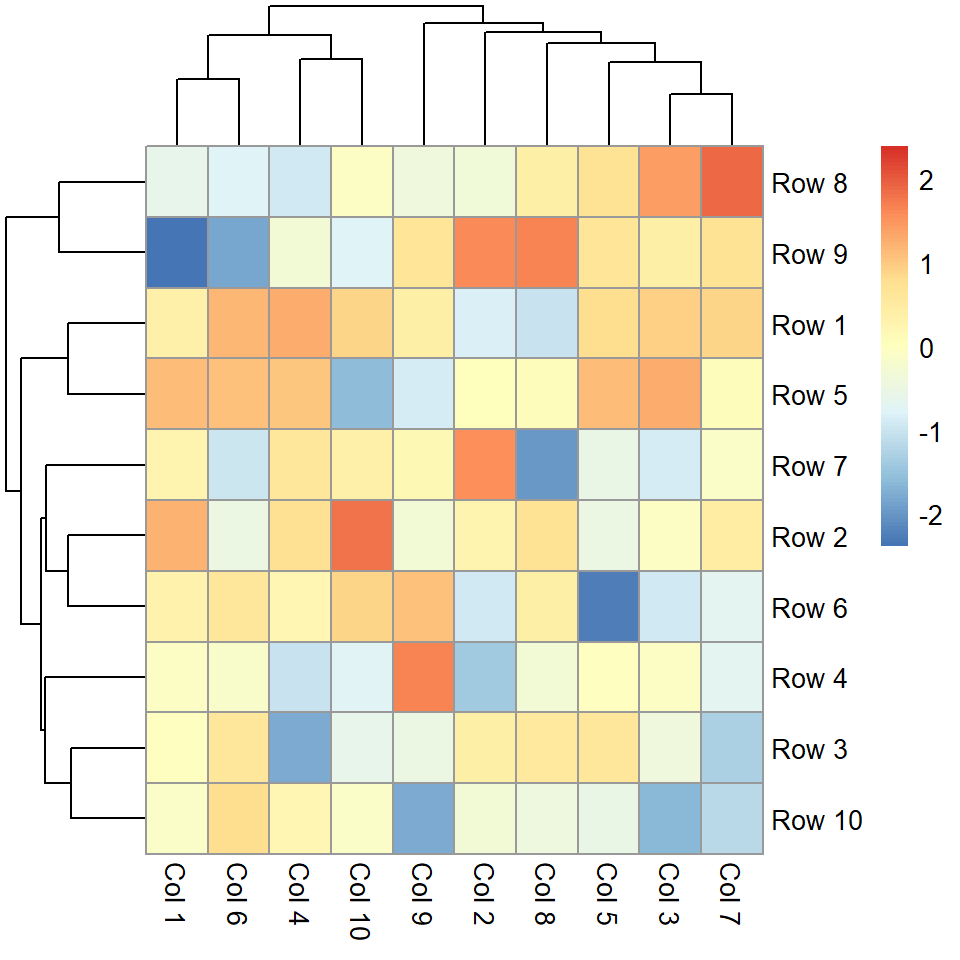Values

If you set `display_numbers = TRUE` the values for each cell will be shown. You can also modify the color and the size of the text.

``````# install.packages("pheatmap")
library(pheatmap)

# Data
set.seed(8)
m <- matrix(rnorm(200), 10, 10)
colnames(m) <- paste("Col", 1:10)
rownames(m) <- paste("Row", 1:10)

# Heat map
pheatmap(m,
display_numbers = TRUE,
number_color = "black",
fontsize_number = 8)``````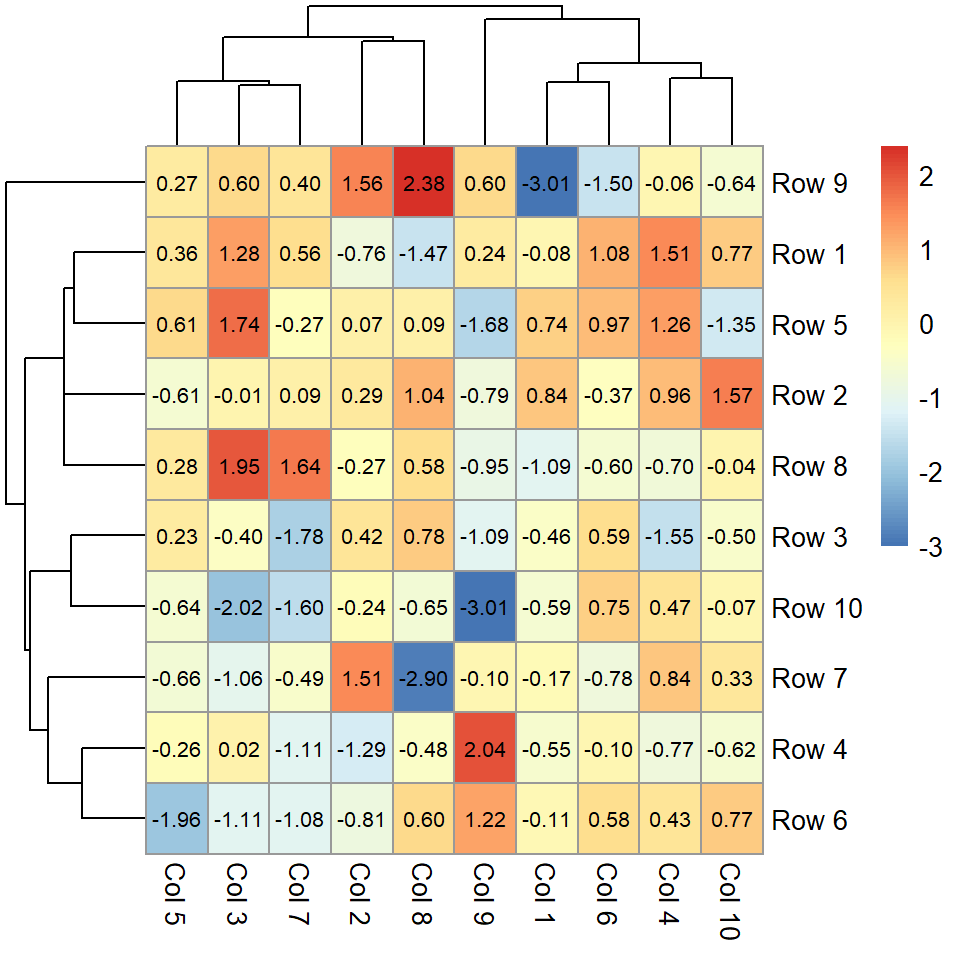Number of clusters

The number of clusters can be changed with `kmeans_k`. If the number of clusters is small you can increase the size of the cells with `cellheight` or `cellwidth`.

``````# install.packages("pheatmap")
library(pheatmap)

# Data
set.seed(8)
m <- matrix(rnorm(200), 10, 10)
colnames(m) <- paste("Col", 1:10)
rownames(m) <- paste("Row", 1:10)

# Heat map
pheatmap(m, kmeans_k = 3, cellheight = 50)``````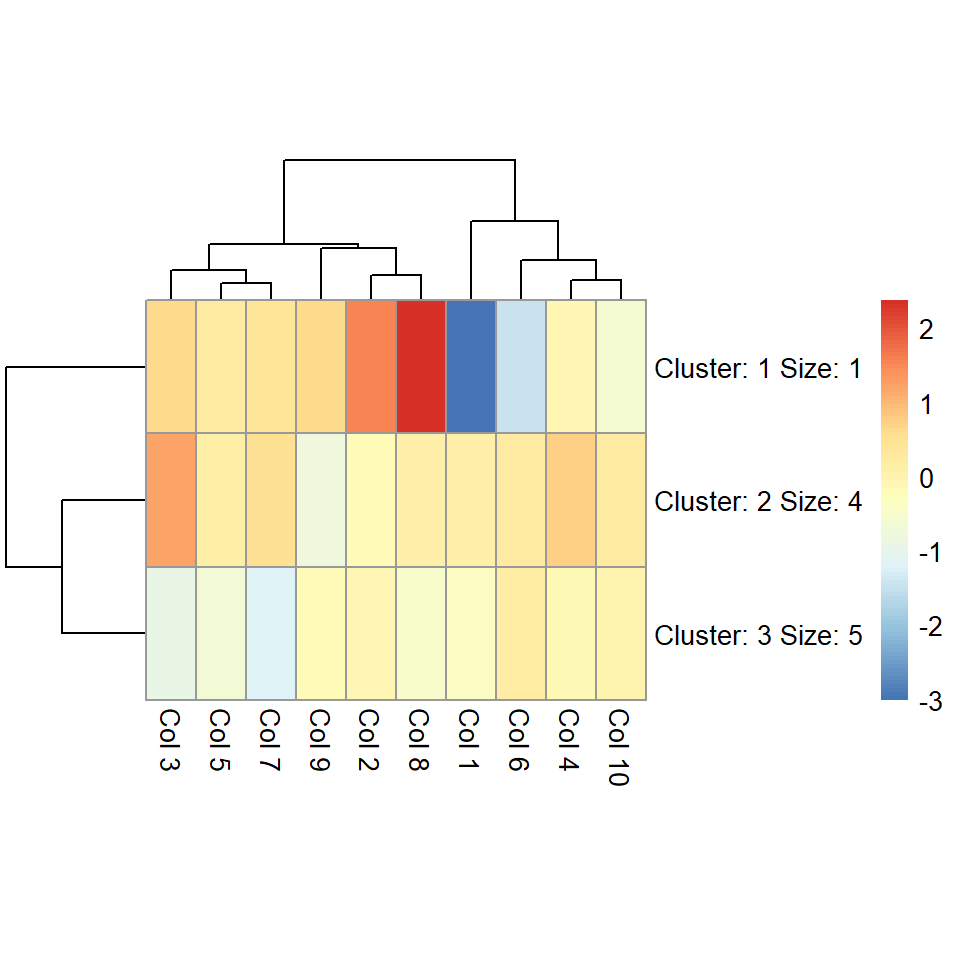## Clustering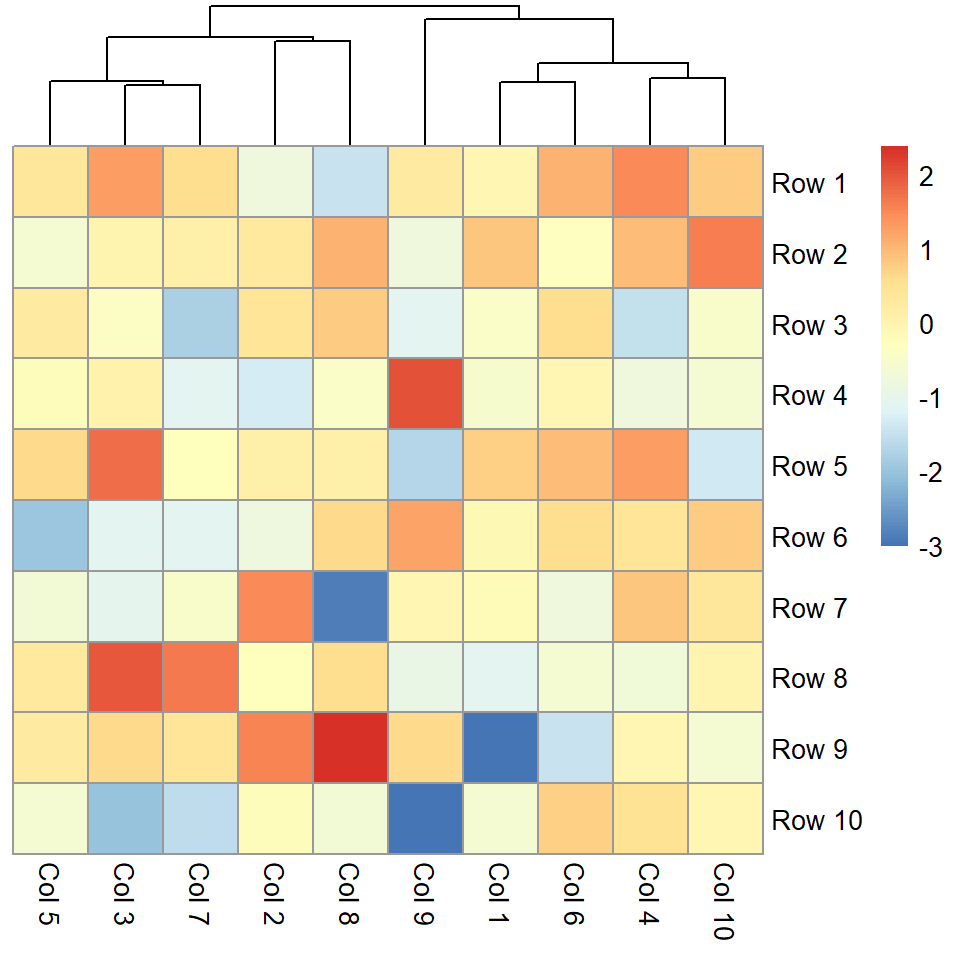Remove rows dendrogram

You can pass a hclust object to the `cluster_rows` argument or set it to `FALSE` to remove the rows dendrogram.

``````# install.packages("pheatmap")
library(pheatmap)

# Data
set.seed(8)
m <- matrix(rnorm(200), 10, 10)
colnames(m) <- paste("Col", 1:10)
rownames(m) <- paste("Row", 1:10)

# Heat map
pheatmap(m, cluster_rows = FALSE)``````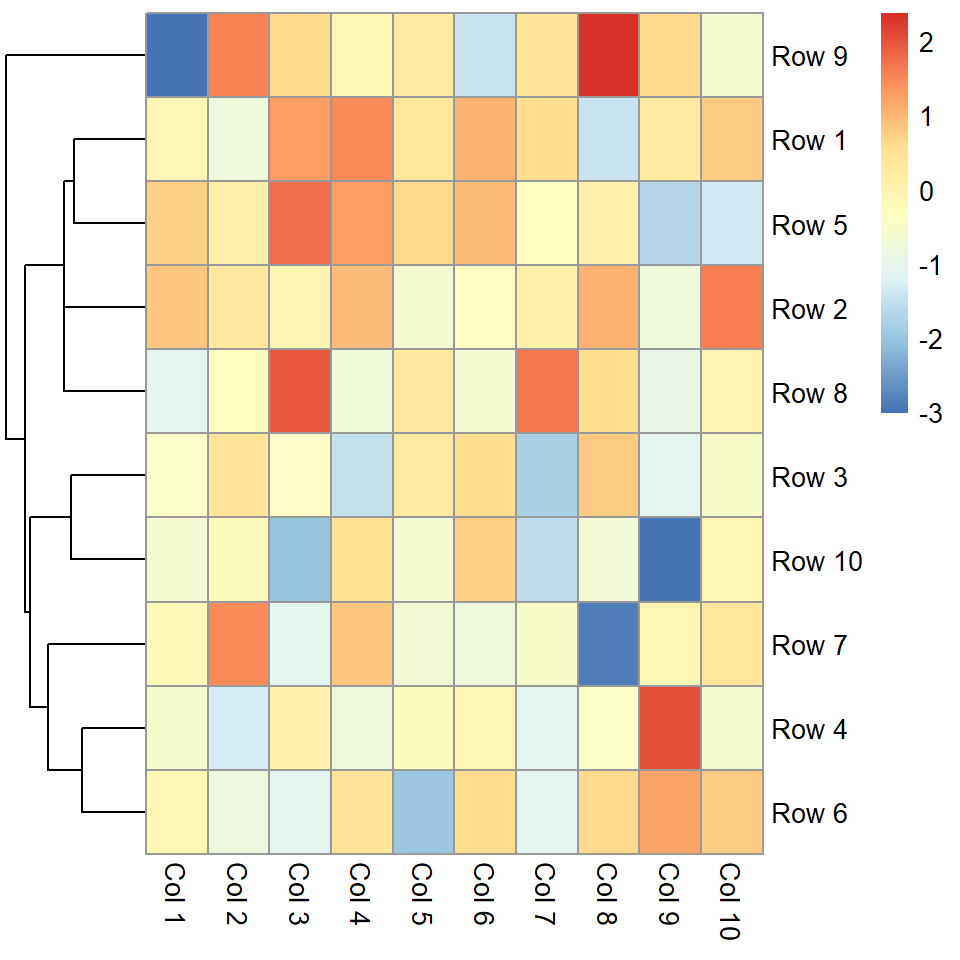Remove columns dendrogram

Equivalently to the previous argument, `cluster_cols` controls how the columns dendrogram should be plotted or if not plot them at all.

``````# install.packages("pheatmap")
library(pheatmap)

# Data
set.seed(8)
m <- matrix(rnorm(200), 10, 10)
colnames(m) <- paste("Col", 1:10)
rownames(m) <- paste("Row", 1:10)

# Heat map
pheatmap(m, cluster_cols = FALSE)``````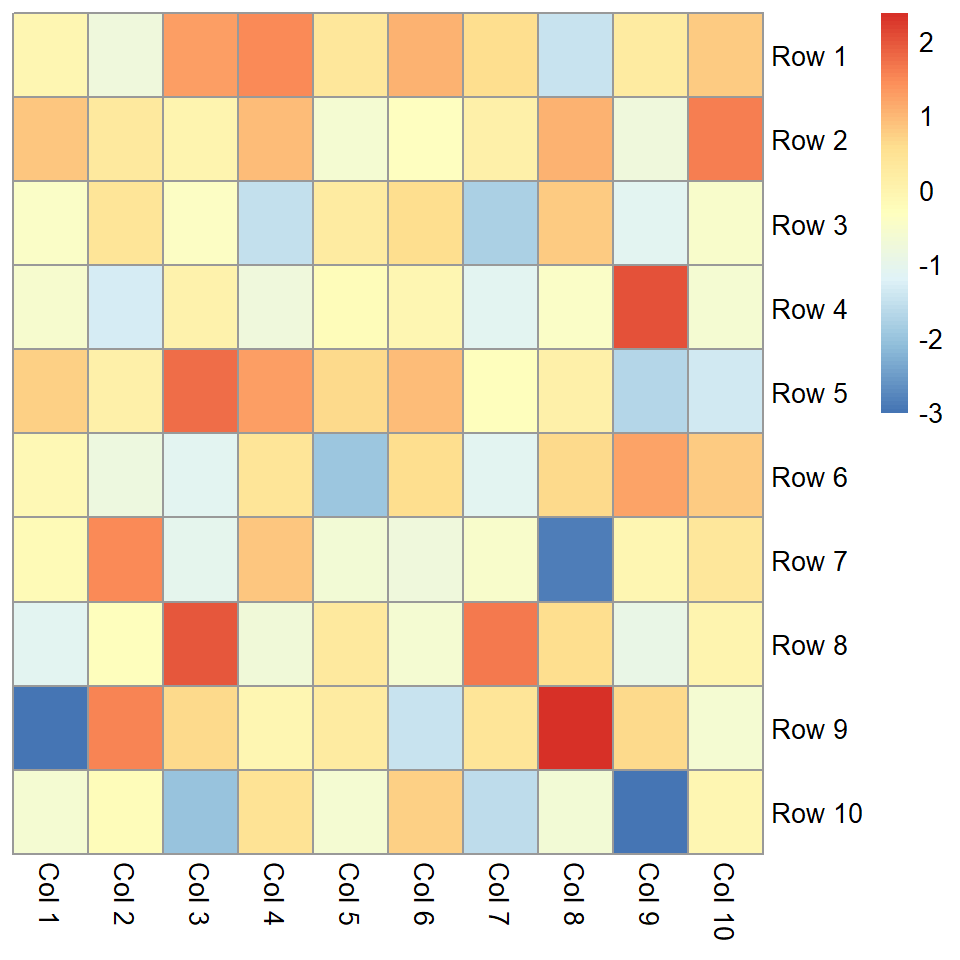Remove dendrograms

It is possible to remove both dendrograms setting `cluster_cols` and `cluster_rows` to `FALSE`.

``````# install.packages("pheatmap")
library(pheatmap)

# Data
set.seed(8)
m <- matrix(rnorm(200), 10, 10)
colnames(m) <- paste("Col", 1:10)
rownames(m) <- paste("Row", 1:10)

# Heat map
pheatmap(m,
cluster_cols = FALSE,
cluster_rows = FALSE)``````

## Color customization

Border color

The `border_color` controls the border of the cells. The default value is `"grey60"`.

``````# install.packages("pheatmap")
library(pheatmap)

# Data
set.seed(8)
m <- matrix(rnorm(200), 10, 10)
colnames(m) <- paste("Col", 1:10)
rownames(m) <- paste("Row", 1:10)

# Heat map
pheatmap(m, border_color = "black")``````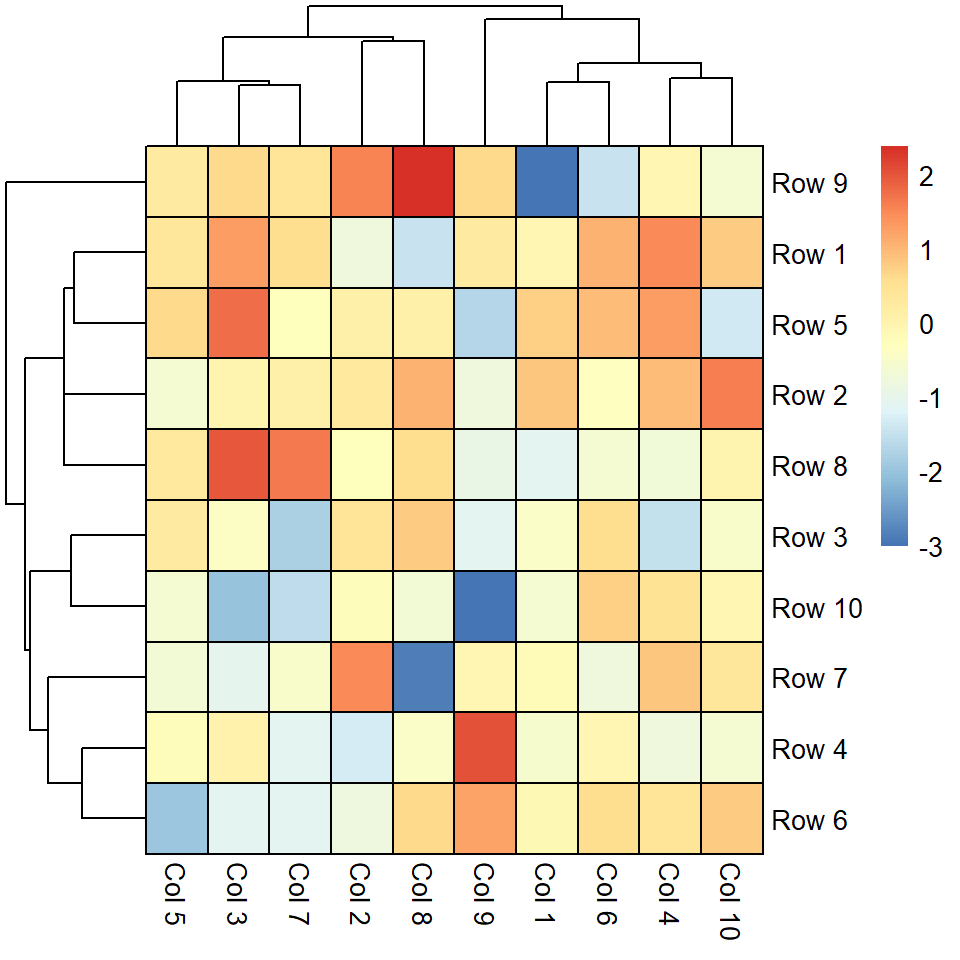Color palette

The default color palette can be changed passing a vector of colors to the `color` argument, as in the example below.

``````# install.packages("pheatmap")
library(pheatmap)

# Data
set.seed(8)
m <- matrix(rnorm(200), 10, 10)
colnames(m) <- paste("Col", 1:10)
rownames(m) <- paste("Row", 1:10)

# Heat map
pheatmap(m, color = hcl.colors(50, "BluYl"))``````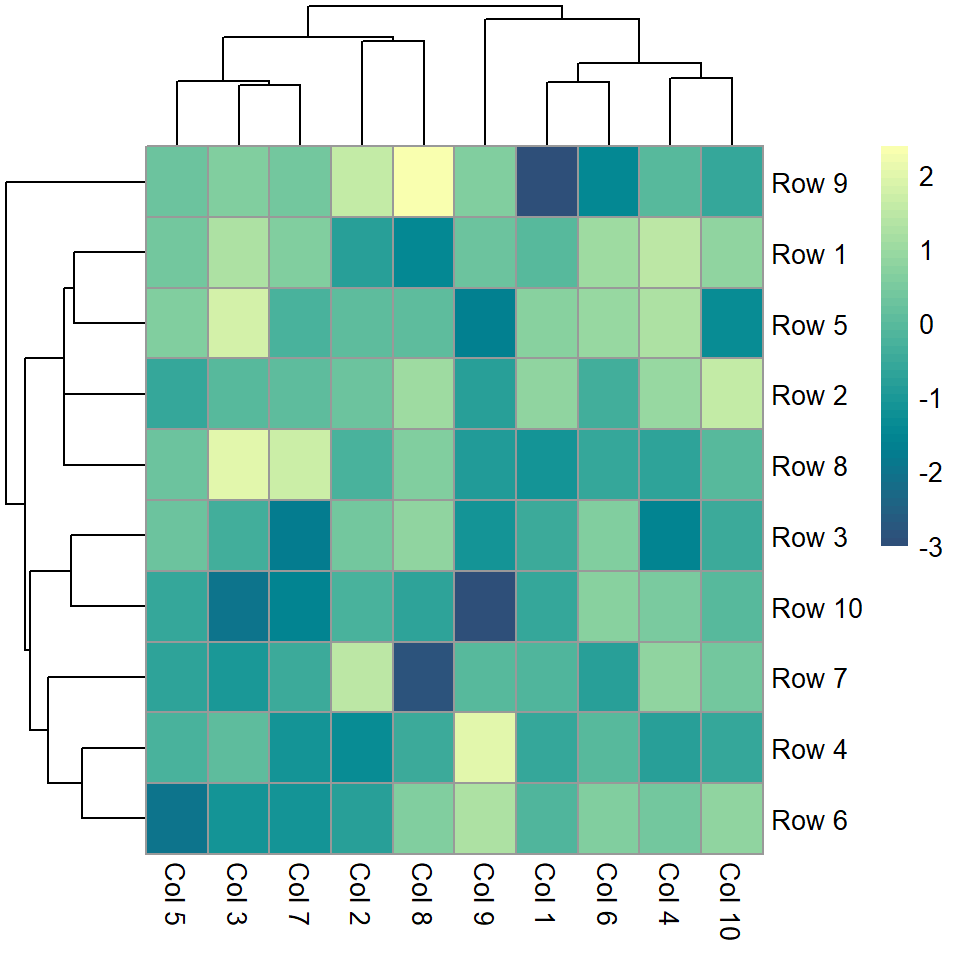## Legend customization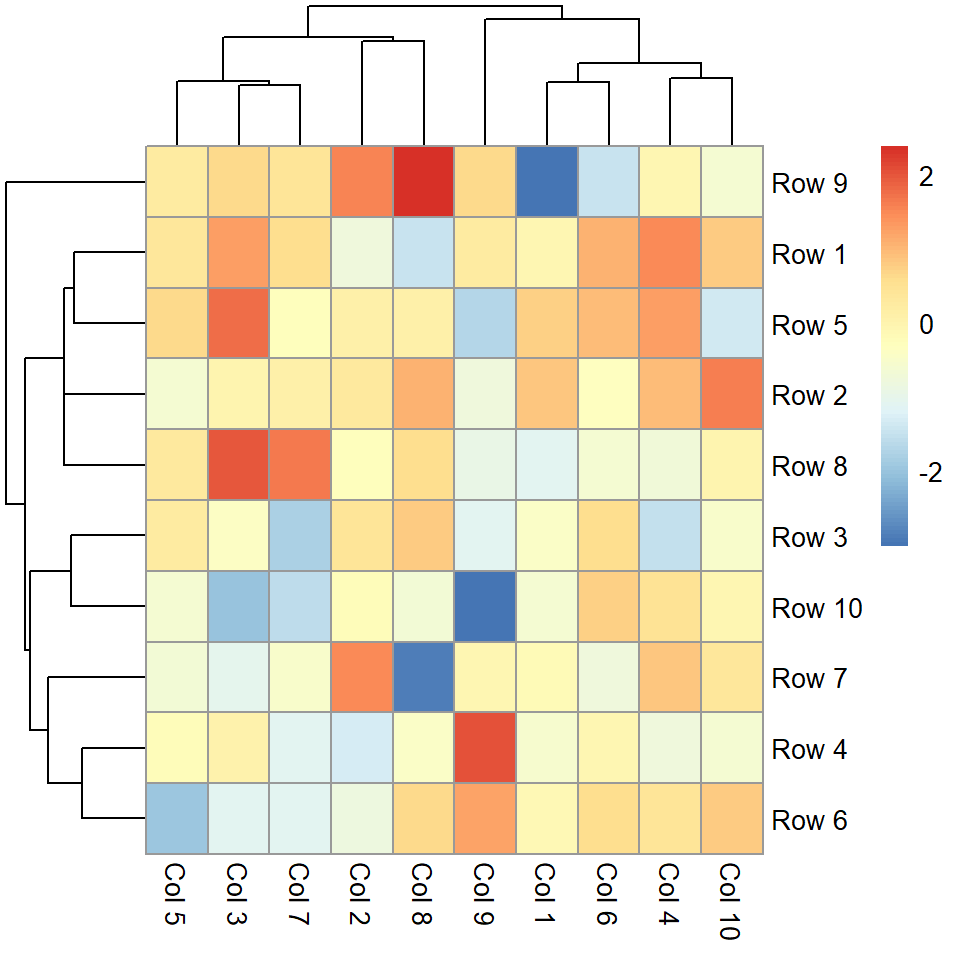Legend breaks

The breaks of the legend can be customized with `legend_breaks`, passing the desired values as a vector.

``````# install.packages("pheatmap")
library(pheatmap)

# Data
set.seed(8)
m <- matrix(rnorm(200), 10, 10)
colnames(m) <- paste("Col", 1:10)
rownames(m) <- paste("Row", 1:10)

# Heat map
pheatmap(m, legend_breaks = c(-2, 0, 2))``````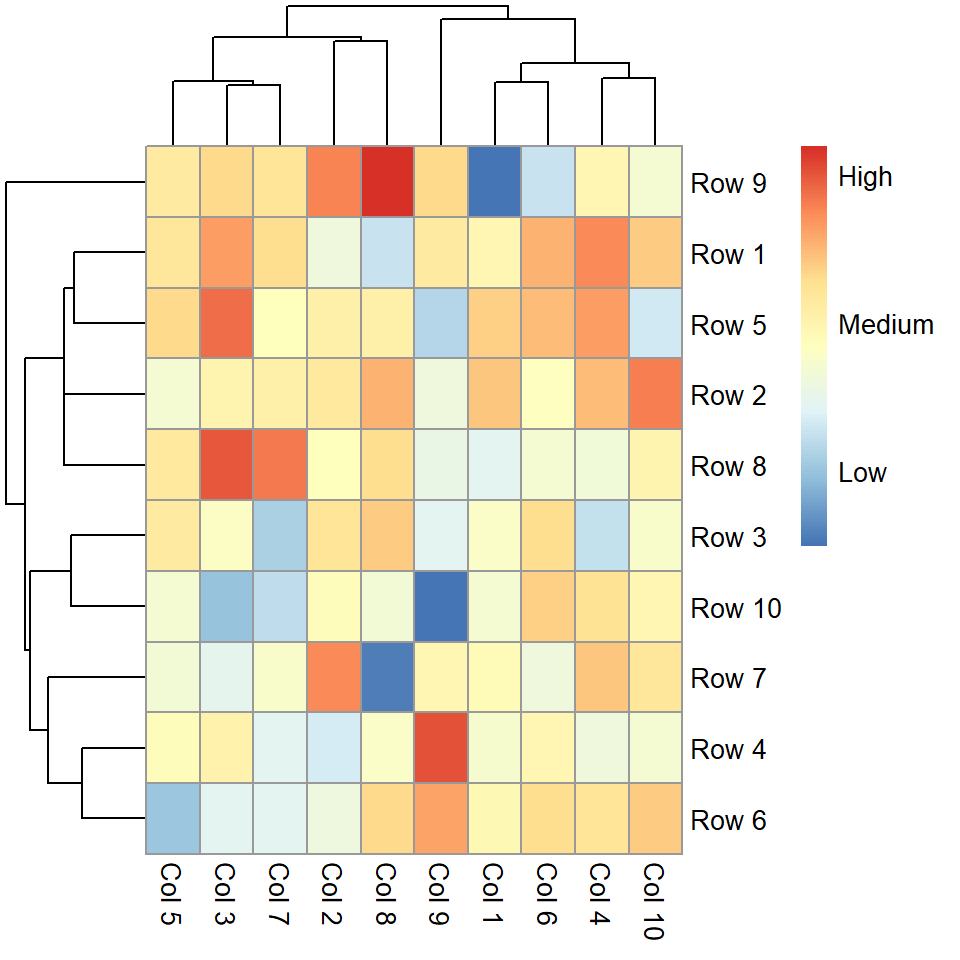Legend labels

If you set the breaks you can also pass a vector of the same length to modify the labels to the `legend_labels` argument.

``````# install.packages("pheatmap")
library(pheatmap)

# Data
set.seed(8)
m <- matrix(rnorm(200), 10, 10)
colnames(m) <- paste("Col", 1:10)
rownames(m) <- paste("Row", 1:10)

# Heat map
pheatmap(m,
legend_breaks = c(-2, 0, 2),
legend_labels = c("Low", "Medium", "High"))``````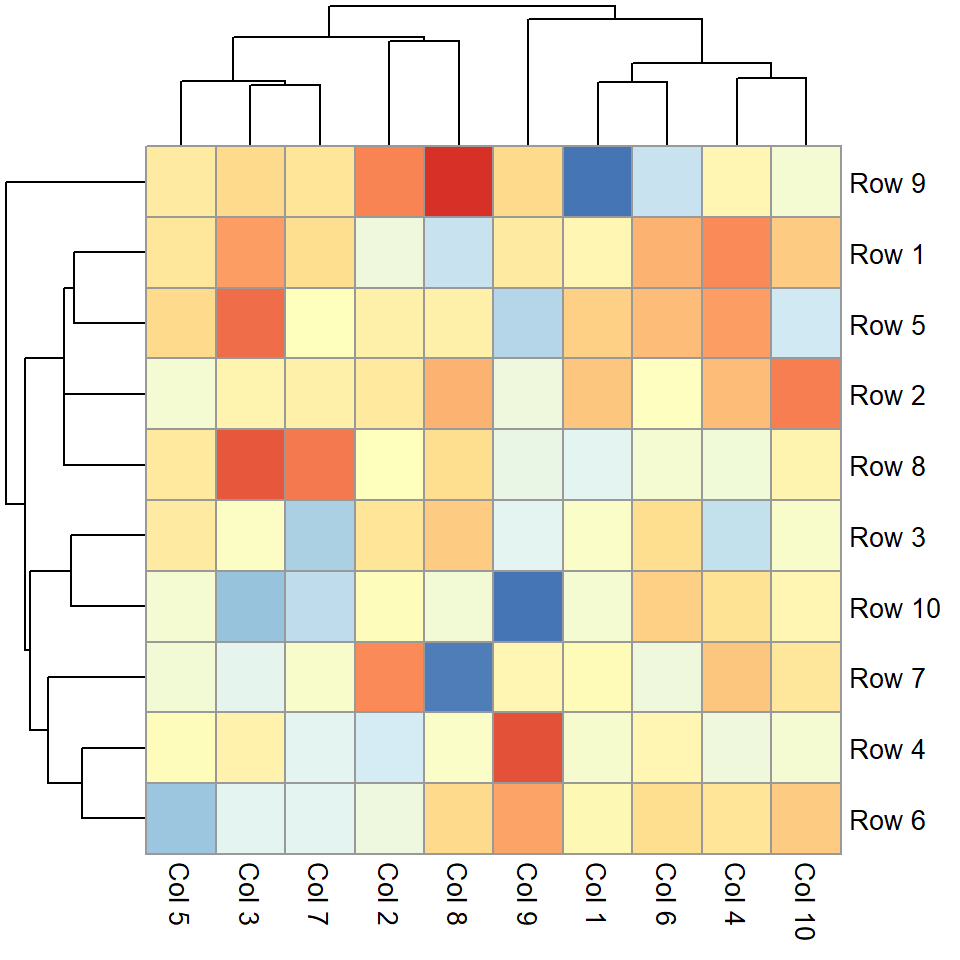Remove the legend

Finally, if you want to get rid of the legend set `legend = FALSE`.

``````# install.packages("pheatmap")
library(pheatmap)

# Data
set.seed(8)
m <- matrix(rnorm(200), 10, 10)
colnames(m) <- paste("Col", 1:10)
rownames(m) <- paste("Row", 1:10)

# Heat map
pheatmap(m, legend = FALSE)``````